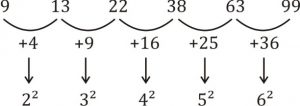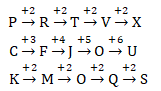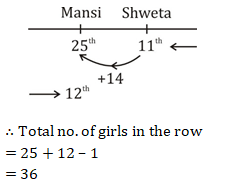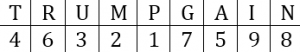# Reasoning [Beginner Level] Quiz For SSC CGL : 25th December

Q1. In the following question, select the odd letter/letters from the given alternatives.
(a) HS
(b) KP
(c) GR
(d) BY

Q2. Arrange the given words in the sequence in which they occur in the dictionary.
2. Real
4. Rather
5. Ratify
(a) 32415
(b) 12543
(c) 45123
(d) 45132

Q3. In the following question, select the missing number from the given series.
9, 13, 22, 38, 63, ?
(a) 96
(b) 99
(c) 84
(d) 90

Q4. A series is given with one term missing. Select the correct alternative from the given ones that will complete the series.
PCK, RFM, TJO, VOQ, ?
(a) XVT
(b) YVT
(c) XUS
(d) ZUS

Q5. In a row of girls, Shweta is 11th from the right end. Mansi is 14th to the left of Shweta. If Mansi is 12th from the left end, then how many girls are there in the row?
(a) 40
(b) 38
(c) 37
(d) 36

Q6. From the given alternatives, select the word which CANNOT be formed using the letters of the given word.
Information
(a) From
(b) Action
(c) Motion
(d) Norm

Q7. In a certain code language, “TRUMP” is written as “46321” and “GRAIN” is written as “76598”. How is “GRUNT” written in that code language?
(a) 23684
(b) 23847
(c) 67834
(d) 76384

Q8. In a certain code language, ‘-‘ represents ‘x’, ‘÷’ represents ‘+’, ‘+’ represents ‘÷’ and ‘x’ represents ‘-‘. Find out the answer to the following question.
2 x 12 ÷ 32 – 5 + 4 = ?
(a) 17
(b) 34
(c) 28
(d) 30

Q9. The following equation is incorrect. Which two signs should be interchanged to correct the equation?
14 + 8 ÷ 16 – 9 x 12 = 10
(a) + and ÷
(b) – and +
(c) + and x
(d) ÷ and x

Q10. If -3%1 = 3, 3%6 = -18 and -7%4 = 28, then find the value of -2%9 = ?
(a) 47
(b) -63
(c) 18
(d) -30

Solutions:

S1. Ans.(c)
Sol. All are pair of opposite letters except GR.

S2. Ans.(d)
Sol. 4. Rather
5. Ratify
2. Real

S3. Ans.(b)
Sol.S4. Ans.(c)
Sol.S5. Ans.(d)
Sol.∴ Total no. of girls in the row
= 25 + 12 – 1
= 36

S6. Ans.(b)
Sol. Action

S7. Ans.(d)
Sol.G R U N T → 7 6 3 8 4

S8. Ans.(d)
Sol. 2×12÷32–5+4
⇒ 2–12+32×5÷4
⇒ 2–12+40
⇒ 42–12
⇒ 30

S9. Ans.(c)
Sol. 14+8÷16–9×12=10
⇒ 14×8÷16–9+12=10
⇒ 7–9+12=10
⇒ 19–9=10
⇒ 10=10

S10. Ans.(c)
Sol. –3×1=–3×(–1)=3
3×6=18×(–1) = – 18
–7×4=–28×(–1)=28
–2×9=–18×(–1)=18

×

Thank You, Your details have been submitted we will get back to you.Join India largest learning distination

What You Will get ?

•Job Alerts
•Daily Quizes
•Subject-Wise Quizes
•Current Affairs
•previous year question papers
•Doubt Solving session

ORJoin India largest learning distination

What You Will get ?

•Job Alerts
•Daily Quizes
•Subject-Wise Quizes
•Current Affairs
•previous year question papers
•Doubt Solving session

ORJoin India largest learning distination

What You Will get ?

•Job Alerts
•Daily Quizes
•Subject-Wise Quizes
•Current Affairs
•previous year question papers
•Doubt Solving session

Enter the email address associated with your account, and we'll email you an OTP to verify it's you.Join India largest learning distination

What You Will get ?

•Job Alerts
•Daily Quizes
•Subject-Wise Quizes
•Current Affairs
•previous year question papers
•Doubt Solving session

## Enter OTP

Please enter the OTP sent to
/6

Did not recive OTP?

Resend in 60sJoin India largest learning distination

What You Will get ?

•Job Alerts
•Daily Quizes
•Subject-Wise Quizes
•Current Affairs
•previous year question papers
•Doubt Solving sessionJoin India largest learning distination

What You Will get ?

•Job Alerts
•Daily Quizes
•Subject-Wise Quizes
•Current Affairs
•previous year question papers
•Doubt Solving session

## Almost there

+91Join India largest learning distination

What You Will get ?

•Job Alerts
•Daily Quizes
•Subject-Wise Quizes
•Current Affairs
•previous year question papers
•Doubt Solving session

## Enter OTP

Please enter the OTP sent to Edit Number

Did not recive OTP?

Resend 60

## By skipping this step you will not recieve any free content avalaible on adda247, also you will miss onto notification and job alerts

Are you sure you want to skip this step?

## By skipping this step you will not recieve any free content avalaible on adda247, also you will miss onto notification and job alerts

Are you sure you want to skip this step?